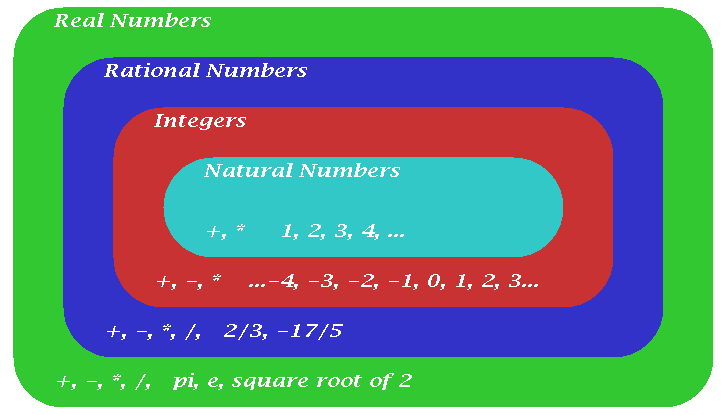# Mathematics 1010 online

## The Number System

On one level this page defines the words we use to distinguish among different types of numbers. However, it also sketches the logical construction underlying the system. This illustrates one of the fundamental principles in doing mathematics.

Introduce concepts in a simple context and then generalize them in such a way that rules and facts that are true in the simple context remain true in the more general context.

### The Natural Numbers.

The natural numbers are

` 1, 2, 3, 4, 5, .... `

More general sets of numbers can be thought of as being constructed to make operations always possible that the more restricted set of natural numbers does not allow. We want to do this in such a fashion that the usual rules of arithmetic remain true. These include, for example:

1. a + b = b + a    The commutative law of addition.
2. a * b = b * a    The commutative law of multiplication.
3. (a + b) + c = a + (b + c)    The associative law of addition.
4. (a * b) * c = a * (b * c)    The associative law of multiplication.
5. (a + b) * c = a * c + b * c    The distributive law.

These laws are true for all and any natural numbers a, b, c. Actually they hold for all numbers we will encounter, but the whole point of building the number system is that we do this in such a way that the above rules remain true.

The distributive law connects multiplication and addition and is the most crucial, and the most misused and misunderstood law in the above list.

## The Integers

The integers include the natural numbers, the number zero, and the negatives of the natural numbers. Thus they are

` ..., -3, -2, -1, 0, 1, 2, 3, .... `

The set of integers can be thought of as having been obtained by expanding the set of natural numbers to make subtraction always possible. Of course we have to define what we mean by the sum, difference, product, and ratio of two integers. This is done in the familiar way with the guiding principle being that the laws listed above remain valid. (That principle for example leads to the requirement that the product of two negative numbers is positive.)

## The Rational Numbers

The rational numbers are ratios of integers (where the divisor is non-zero), such as 3/4, -2/17, etc. Note that the integers are included among the rational numbers, for example, the integer 3 can be written as 3/1, or even 6/2 .

The result of adding, subtracting, multiplying, or dividing rational numbers (so long as we don't divide by zero) is another rational number. We say that the set of rational numbers is closed under addition, subtraction, multiplication, and division.

## The Real Numbers

It turns out that the rational numbers are not enough to describe the world. Consider, for example, a right triangle whose two short sides are each 1 foot long. By the Pythagorean Theorem, the long side, the hypotenuse, has a length whose square equals 2. That length is referred to as the square root of 2.

It can be shown that there is no rational number whose square equals 2. Hence the number system needs to be extended once more. For our purposes a real number is a decimal expression whose digits may or may not terminate or repeat. It can also be shown that a real number is rational if and only if its digit repeat or terminate. Real numbers that aren't rational are irrational

## Hierarchy of Numbers

One of the harder things to understand and appreciate about numbers is that they form a hierarchy. Just like all trout are fish, all fish are vertebrates, and all vertebrates are animals, all natural numbers are integers, all integers are rational numbers, and all rational numbers are real numbers. On the other hand, just like some fish aren't trout, some real numbers aren't rational, some rational numbers aren't integer, and some integers aren't natural numbers. For some reason this tends to be very confusing, but it's just like saying that all women are people, but some people aren't women. (The same goes for men, of course, and then there are kids too.) Never mind the people, but you need to understand this language, and be prepared to give examples of the various types of numbers. The hierarchy of the number system is illustrated in this Figure:## Complex Numbers

Later in this course we will learn about a yet more general set of numbers, the complex numbers.